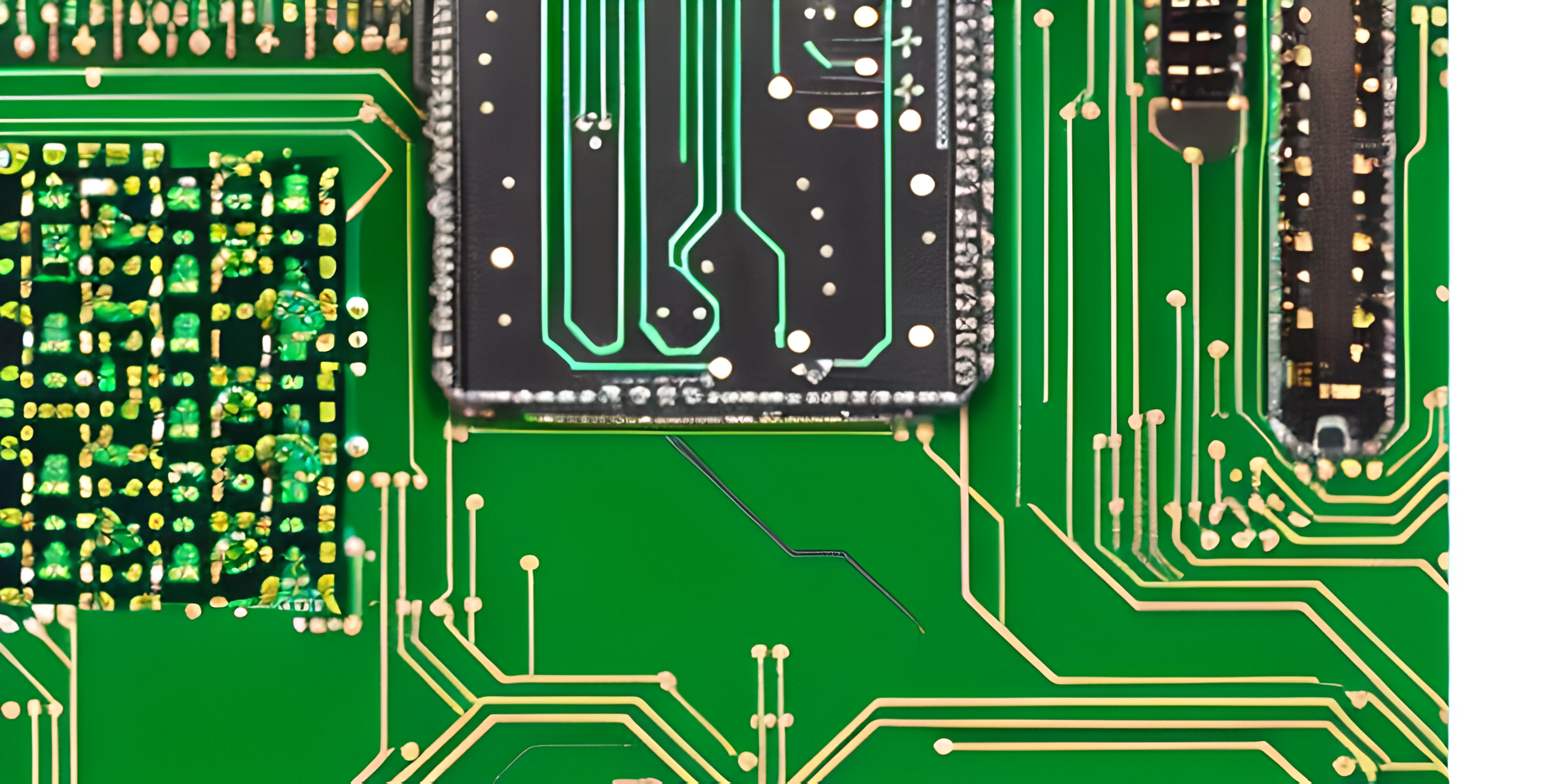# x86 Assembly: Pointers and Arrays in NASMNote: this page has been created with the use of AI. Please take caution, and note that the content of this page does not necessarily reflect the opinion of Cratecode.

Pointers and arrays are two essential concepts in programming, and they're just as important in x86 Assembly when using the Netwide Assembler (NASM). In this exciting journey, we'll explore the world of pointers and arrays in x86 Assembly, uncovering their mysteries and learning how to wield them like a pro.

## Pointers in x86 Assembly

A pointer is a variable that stores the memory address of another variable or value, making it incredibly useful for working with memory or referencing large data structures. In x86 Assembly, pointers are usually stored in registers, and we can manipulate them using specific instructions.

### Creating a Pointer

To create a pointer in x86 Assembly, we need to load the address of a variable or value into a register. Let's say we have a variable called `myVar`. Here's how to create a pointer to `myVar`:

``````; Declare a variable
myVar db 42

; Create a pointer to myVar
mov eax, myVar``````

Now, `eax` contains the address of `myVar`. We can say that `eax` is a pointer to `myVar`.

### Dereferencing a Pointer

To access the value stored at the address pointed to by a pointer, we need to "dereference" the pointer. In x86 Assembly, we use the square brackets `[]` to dereference pointers. Let's see how to do this with our `eax` pointer:

``````; Create a pointer to myVar
mov eax, myVar

; Dereference the pointer and store the value in ebx
mov ebx, [eax]``````

Now, `ebx` contains the value `42`, which is the value stored at the memory location pointed to by `eax`.

## Arrays in x86 Assembly

An array is a collection of elements (usually of the same type) stored sequentially in memory. In x86 Assembly, we can create arrays using the `db`, `dw`, or `dd` directives, depending on the size of the elements.

### Creating an Array

Let's create an array of bytes called `myArray`:

``````; Declare an array of bytes
myArray db 1, 2, 3, 4, 5``````

Now, we have an array of five elements, each containing a byte of data.

### Accessing Array Elements

To access an array element, we need to calculate its memory address using the base address of the array and the index of the element. The formula is:

``element_address = base_address + (index * element_size)``

Here's an example of accessing the third element (index 2) of `myArray`:

``````; Load the base address of myArray into eax
mov eax, myArray

; Calculate the address of the third element (index 2)

; Load the value of the third element into ebx
mov ebx, [eax]``````

Now, `ebx` contains the value `3`, which is the third element of `myArray`.

And there you have it! You've unlocked the secrets of pointers and arrays in x86 Assembly using NASM. With these powerful tools in your arsenal, you're well-equipped to tackle the challenges of low-level programming. Happy coding!

## FAQ

### What are pointers and arrays in x86 Assembly NASM?

Pointers are variables that store memory addresses, while arrays are collections of elements of the same data type, stored in contiguous memory locations. In x86 Assembly using NASM, pointers and arrays are used to efficiently manipulate and access data in memory.

### How do I declare an array in x86 Assembly NASM?

To declare an array in x86 Assembly using NASM, you can use the `db`, `dw`, or `dd` directives to define byte, word, or double-word arrays, respectively. For example:

``myArray db 1, 2, 3, 4, 5 ; byte array``

### How can I access elements of an array in x86 Assembly NASM?

To access elements of an array in x86 Assembly using NASM, you can use registers to store the base address of the array and then add an offset to access the desired element. Here's an example:

``````mov eax, [myArray]       ; Load the base address of the array into eax
add eax, 2               ; Add an offset of 2 to access the third element
mov al, [eax]            ; Load the value of the third element into the al register``````

### How do I work with pointers in x86 Assembly NASM?

To work with pointers in x86 Assembly using NASM, you can use registers to store memory addresses and then access the data at those addresses using brackets. Here's an example:

``````mov eax, myArray         ; Load the base address of the array into eax (pointer)
mov al, [eax]            ; Load the value at the memory address in eax into al``````

### What is pointer arithmetic, and how do I perform it in x86 Assembly NASM?

Pointer arithmetic refers to operations that modify the memory address stored in a pointer, such as adding or subtracting an offset. In x86 Assembly using NASM, you can perform pointer arithmetic using `add`, `sub`, or other arithmetic instructions. Here's an example:

``````mov eax, myArray         ; Load the base address of the array into eax (pointer)